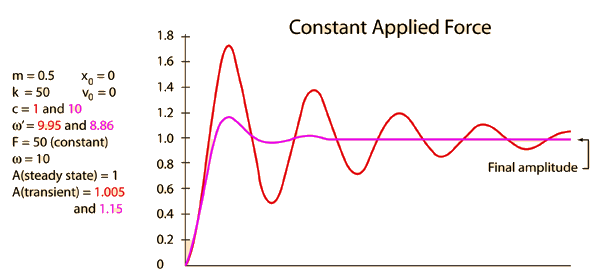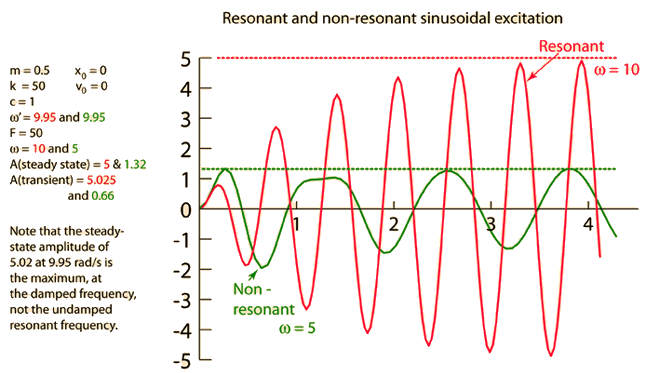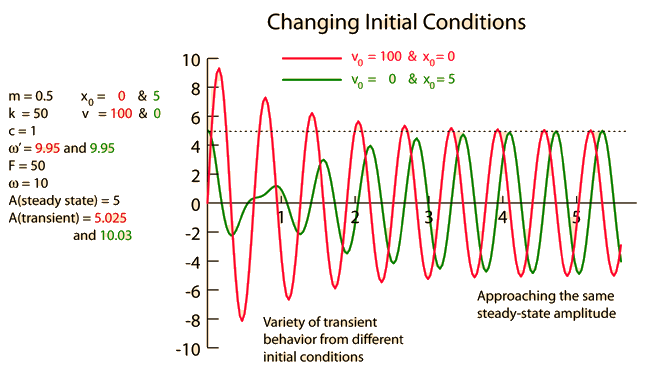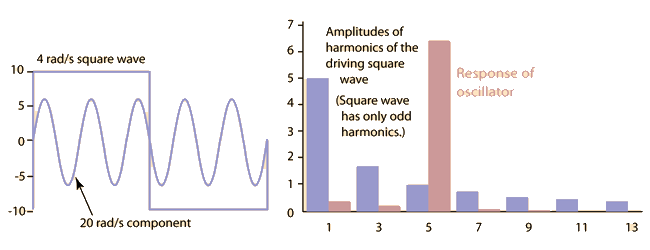# Driven Oscillator Examples

If a damped oscillator is driven by an external force, the solution to the motion equation has two parts, a transient part and a steady-state part, which must be used together to fit the physical boundary conditions of the problem.

## Constant applied force

If a constant force is applied to a damped oscillator, it will stretch out to a final position determined by its spring constant. However, depending upon the initial conditions, it may oscillate about that final position and then settle down to the final position. This is the practical implication of the transient solution to the motion equations.

## Resonant excitation

If a sinusoidal driving force is applied at the resonant frequency of the oscillator, then its motion will build up in amplitude to the point where it is limited by the damping forces on the system. If the damping forces are small, a resonant system can build up to amplitudes large enough to be destructive to the system. Such was the famous case of the Tacoma Narrows Bridge, which was blown down by the wind when it responded to a component in the wind force which excited one of its resonant frequencies.

## Changing initial conditions

The effect of the transient solution on the behavior of a damped oscillator is strongly dependent upon the initial conditions. Although a driven system will ultimately settle down to a behavior determined by the driving force (steady-state solution), the early part of the motion can show a lot of variety.

## Complex exciting forces

It is the nature of a resonant system to respond strongly to influences which have frequencies close to its resonant frequency. If a complex exciting force is applied, i.e., one which will have many frequency components, then the system will tend to pick out the components which are close to its resonant frequency. The example given is that of a square-wave driving force

 Driven oscillator Transient solution Steady-state solution

Index

Periodic motion concepts

Driven oscillator

 HyperPhysics***** Mechanics R Nave
Go Back

# Driven Oscillator Example: Constant Applied Force

If a constant force is applied to a damped oscillator, it will stretch out to a final position x = F/k determined by its spring constant. However, depending upon the initial conditions, it may oscillate about that final position and then settle down to the final position. This is the practical implication of the transient solution to the motion equations. This illustration shows transient behavior for damping coefficients which differ by a factor of 10.Index

Periodic motion concepts

Driven oscillator

 HyperPhysics***** Mechanics R Nave
Go Back

# Driven Oscillator Example

If a sinusoidal driving force is applied at the resonant frequency of the oscillator, then its motion will build up in amplitude to the point where it is limited by the damping forces on the system. If the damping forces are small, a resonant system can build up to amplitudes large enough to be destructive to the system. Such was the famous case of the Tacoma Narrows Bridge, which was blown down by the wind when it responded to a component in the wind force which excited one of its resonant frequencies.Since a resonant excitation will continue to contribute energy to the system at its natural frequency, the amplitude of oscillation will continue to grow until that energy is dissipated by the damping forces, or until something destructive happens to the system. There are many stories about mechanical resonances being produced by pieces of mechanical equipment which produce periodic vibrations, like air-compressors.

Index

Periodic motion concepts

Driven oscillator

 HyperPhysics***** Mechanics R Nave
Go Back

# Driven Oscillator Example

The effect of the transient solution on the behavior of a damped oscillator is strongly dependent upon the initial conditions. Although a driven system will ultimately settle down to a behavior determined by the driving force (steady-state solution), the early part of the motion can show a lot of variety.Index

Periodic motion concepts

Driven oscillator

 HyperPhysics***** Mechanics R Nave
Go Back

# Driven Oscillator Example

It is the nature of a resonant system to respond strongly to influences which have frequencies close to its resonant frequency. If a complex exciting force is applied, i.e., one which will have many frequency components, then the system will tend to pick out the components which are close to its resonant frequency. The example given is that of a square-wave driving force

The Fourier analysis of a square wave yields odd harmonics with relative amplitude A1/n . The excitation of an oscillator of natural frequency 20 rad/s with a 4 rad/s square wave gives maximum excitation at n=5, even though the driving amplitude is down by a factor of 5.Index

Periodic motion concepts

Driven oscillator

 HyperPhysics***** Mechanics R Nave
Go Back